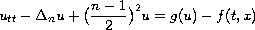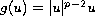Seventh Mississippi State - UAB Conference on Differential Equations and Computational Simulations. Electron. J. Diff. Eqns., Conference 17 (2009), pp. 95-105.

### Infinitely many periodic solutions of nonlinear wave equations on $S^n$ Jintae Kim

Abstract:
The existence of time periodic solutions of nonlinear wave equationson-dimensional spheres is considered. The corresponding functional of the equation is studied by the convexity in suitable subspaces, minimax arguments for almost symmetric functional, comparison principles and Morse theory. The existence of infinitely many time periodic solutions is obtained whereand the non-symmetric perturbationis not small.

Published April 15, 2009.
Math Subject Classifications: 20H15, 20F18, 20E99, 53C55.
Key Words: Minimax theory; Morse index; critical points.

Show me the PDF file (245 KB), TEX file, and other files for this article.Jintae Kim Department of Mathematics, Tuskegee University Tuskegee, AL 36088, USA email: jtkim@tuskegee.edu